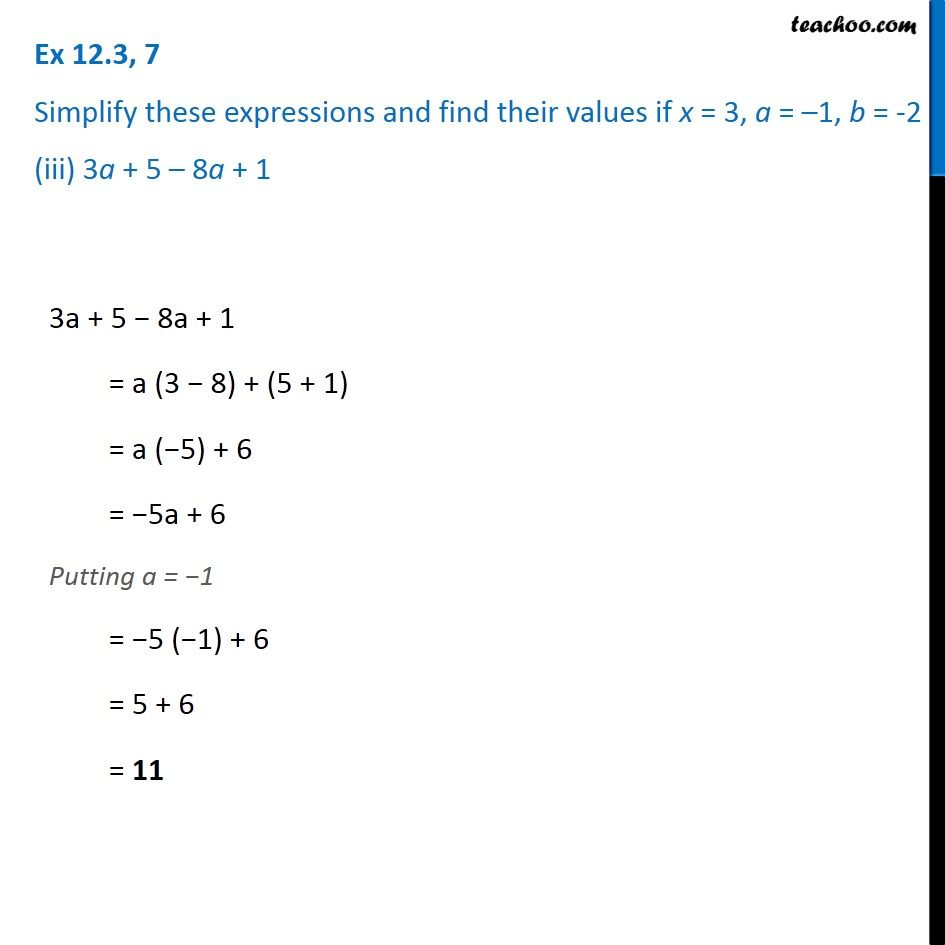Ex 12.3

Chapter 12 Class 7 Algebraic Expressions
Serial order wiseGet live Maths 1-on-1 Classs - Class 6 to 12

### Transcript

Ex 12.3, 7 Simplify these expressions and find their values if x = 3, a = –1, b = -2 (iii) 3a + 5 – 8a + 1 3a + 5 − 8a + 1 = a (3 − 8) + (5 + 1) = a (−5) + 6 = −5a + 6 Putting a = −1 = −5 (−1) + 6 = 5 + 6 = 11